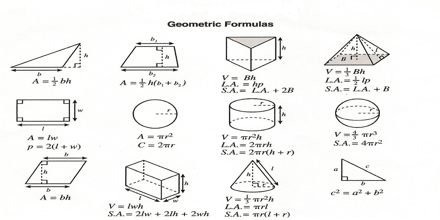Mathematic

# Formulas for GeometryMain objective of this lecture is to present on Formulas for Geometry. Mathematicians have created formulas to save you time. But, they always change the letters of the formulas to scare you. Squares, rectangles and parallelograms area are almost the same (LxW). Triangles are ½ cause your missing ½. Circles have fancy names, but just follow the formula. here briefly describe on formulas for geometry, related to area and perimeter of triangles, rectangles, cercles, sectors, and volume of sphere, cone, cylinder are presented.